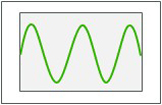# Crystal UnitsBasic Knowledge

Description of technical reference of crystal units

[Do you encounter issues such as these?]Issues such as these can be caused when “oscillation stops” or “the frequency deviates significantly” in an oscillation circuit employing a crystal unit.To prevent such problems and ensure stable oscillation, an optimized circuit design is essential.

Here you can view five videos on circuit evaluation from Murata Manufacturing, the experts in the field.

##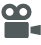Video Seminars (Five in Total)

Session 1: Roles of circuit elements
This seminar describes the roles of the various circuit elements and explains the oscillation characteristics you will need to check in order to select the optimal circuit constants.

Session 2: Measuring the oscillation margin
This seminar describes an oscillation margin, shows how to calculate it, and how to measure the negative resistance.

Session 3: Measuring the drive level
This seminar describes a drive level, shows examples of how to calculate it, and explains how to measure it.

Session 4: Measuring the oscillation frequency
This seminar describes oscillation frequency and how to measure it, and also explains oscillation frequency correlation and gives related hints.

Session 5: Relationship between oscillation characteristics and constants
This seminar covers the basic workflow for determining the relationship between oscillation characteristics and constants and performing circuit evaluation, explains the relationship between oscillation characteristics and constants, shows how the various characteristics change when the circuit constants are altered, and describes the procedure of basic circuit evaluation.

##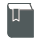Basic knowledge

Explains frequently used technical terms related to crystal units, such as resonance frequency (fr), equivalent circuit, and pulling sensitivity.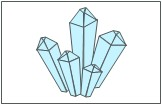Explains oscillation margin and how to calculate (determine) it, as well as how to measure or make a quick check of the negative resistance, etc.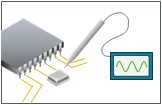Describes drive level, explains how to calculate (determine) it, and gives an example of how to measure drive level, etc.Describes what an oscillation frequency is, shows how to calculate and measure the oscillating frequency (frequency counter, non-contact and spectrum analyzer, non-contact), etc.Describes oscillation amplitude, shows what is required to measure it, and presents notes on things to consider, etc.